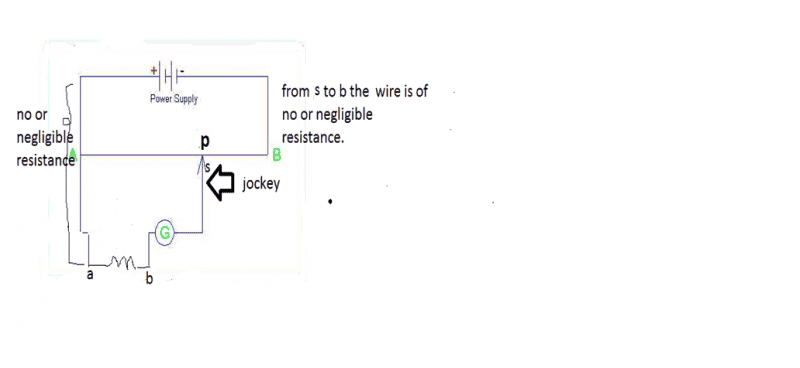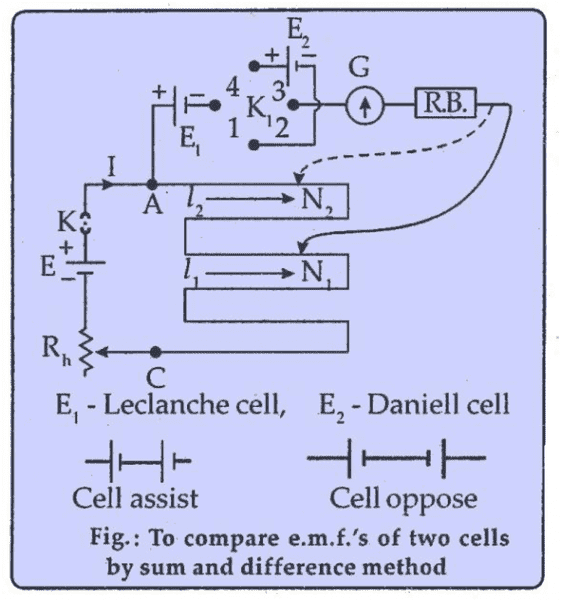# Potentiometer and galvanometer experiment

## Homework Statement

Zero deflection in galvanometer

## Homework Equations

If potential difference across between two points between which galvanometer is kept is zero galvanometer shows zero deflection.

## The Attempt at a Solution

But my doubt starts from this video at 3:33 to 3:44 teacher says that potential at p is same as potential at b,provided that connecting wires (represented in green color in video)are having no resistance.I think it can only happen if internal resistance of galvanometer is same as resistance between length AP.So that potential at p is same as potential at b,and galvanometer will show zero deflection.Am i right?

gneill
Mentor
Gracy, very few homework helpers will be interested in viewing nearly 4 minute of extraneous material in order to suss out the details of your question. How about you post a circuit diagram and your own analysis of the problem with some details about your concerns?

Also, what does "potential difference across between two points between which galvanometer is kept is zero galvanometer shows zero deflection" mean? My English language parser has failed me on this one, and I'm a native English speaker. Can you clarify your query?

Gracy, very few homework helpers will be interested in viewing nearly 4 minute of extraneous material in order to suss out the details of your question. How about you post a circuit diagram and your own analysis of the problem with some details about your concerns?

Also, what does "potential difference across between two points between which galvanometer is kept is zero galvanometer shows zero deflection" mean? My English language parser has failed me on this one, and I'm a native English speaker. Can you clarify your query?
It's not about English language.It is just because of my my haste.I didn't check my question once before posting.Actually i am very nervous about my upcoming test.Sorry,I'll do the needed correction.But i don't think it was too difficult to interpret .I am rewriting that sentence
'If potential difference across two point is zero(or if two points are at same potential) ,galvanometer shows zero deflection'.So overall i had made 2 mistakes i used both( across and between ).And second mistake i made was in order to explain the conditions clearly I included 'between which galvanometer is kept'I should have thought that if i really need to explain correctly and clearly i need to post a circuit diagram .Words(that too written in disturbed state of mind)are not going to make up for circuit diagrams which are backbone of electronics.

Last edited:

## Homework Statement

Zero deflection in galvanometer

## Homework Equations

If potential difference across two points is zero ,galvanometer shows zero deflection.

## The Attempt at a Solution

But my doubt starts from this video at 3:33 to 3:44 teacher says that potential at p is same as potential at b,provided that connecting wires (represented in green color in video)are having no resistance.I think it can only happen if internal resistance of galvanometer is same as resistance between length AP.So that potential at p is same as potential at b,and galvanometer will show zero deflection.Am i right?

NascentOxygen
Staff Emeritus
We should download a 10:24 youtube video in lieu of a single screen-capture jpg?No thanks. :(a is at same potential as A(as connecting wire is of negligible resistance.And p is particular point is found to be at same potential as b because galvanometer shows zero deflection.My question is why p is a particular point to be at same potential as b,i mean if from s to b the connecting wire is of negligible resistance than any point between length AB will have same potential as b.I think it is because galvanometer internal resistance.So that means galvanometer resistance is same as resistance present in length Ap.Am i right?

We should download a 10:24 youtube video in lieu of a single screen-capture jpg?No thanks. :(
I didn't understand.But it is certainly not answer of my question.

NascentOxygen
Staff Emeritus
a is at same potential as A(as connecting wire is of negligible resistance.And p is particular point is found to be at same potential as b because galvanometer shows zero deflection.My question is why p is a particular point to be at same potential as b,i mean if from s to b the connecting wire is of negligible resistance than any point between length AB will have same potential as b.I think it is because galvanometer internal resistance.So that means galvanometer resistance is same as resistance present in length Ap.Am i right?

The photo is welcome.

AB is a resistance wire; it has resistance all along its length. By sliding the jockey along it, you can set point P to have any potential between the battery's (+) potential and ground. (It might help if you showed the wire AB of a different thickness or color, so as to clearly distinguish it from hookup wire.)
What is that element between points a and b?

Last edited:
What is that element between points a and b?[/QUOTE]
It is resistance.

NascentOxygen
Staff Emeritus
What is that element between points a and b?
It is resistance.
I think you should be considering there is a voltage source between points a and b, not a resistance. The potentiometer allows you to determine what voltage that voltage source is. That is the purpose of a potentiometer. The galvanometer indicates when the voltage at the sliding jockey equals the voltage of the unknown voltage source Vab.

If the unknown voltage source has resistance, too, that doesn't matter, because when the galvanometer indicates a balance point, there is zero current from/to that voltage source, so there is no potential drop across any internal resistance.

It's not surprising you were confused, when you thought it was just a resistance.Last edited:
•gracy
ehild
Homework Helper
It's not surprising you were confused, when you thought it was a resistance.It was drawn a resistance there but it was said that the potential difference across that resistance is measured. That resistance was part of a circuit, containing voltage source. A very confusing video !

•gracy
NascentOxygen
Staff Emeritus
Ah, so the seed of confusion was set in the video.

All sorted now, I hope.

The photo is welcome.

AB is a resistance wire; it has resistance all along its length. By sliding the jockey along it, you can set point P to have any potential between the battery's (+) potential and ground. (It might help if you showed the wire AB of a different thickness or color, so as to clearly distinguish it from hookup wire.)
What is that element between points a and b?
Have you also assumed resistance free or of negligible resistance conducting wire?

NascentOxygen
Staff Emeritus
Have you also assumed resistance free or of negligible resistance conducting wire?
That is always a default assumption, in any circuit. But based on what I wrote, you yourself should now be able to highlight those connecting wires where extra resistance will make no difference to the result. What is the key factor to identifying those wires?

That is always a default assumption, in any circuit. But based on what I wrote, you yourself should now be able to highlight those connecting wires where extra resistance will make no difference to the result. What is the key factor to identifying those wires?
I think if two points are at same potential than no current will flow ,so extra resistance will not matter.

NascentOxygen
Staff Emeritus
I think if two points are at same potential than no current will flow ,so extra resistance will not matter.
That's correct. So you just need to now identify those two points that will have equal potential.

That's correct. So you just need to now identify those two points that will have equal potential.
A and a ,p (if zero deflection point is shown by galvanometer at point p)and b.But i am not getting one thing if two points have resistance between them then how can they be at equal potential as presence of resistance results in potential drop.

Last edited:
NascentOxygen
Staff Emeritus
A and a ,p (if zero deflection point is shown by galvanometer at point p)and b.But i am not getting one thing if two points have resistance between them then how can they be at equal potential as presence of resistance results in potential drop.
At balance, how much current is flowing through the galvanometer and its connecting wires?

Perhaps you are still not appreciating that between a and b there is a battery of some to-be-determined potential? So when the potentiometer slider is moved to the exact same potential as that of the battery, those voltages will be equal.

I have a question.,Why there is always one null deflection point?Is it not possible that we slide jockey but there is no such point obtained where galvanometer shows zero deflection?

NascentOxygen
Staff Emeritus
I have a question.,Why there is always one null deflection point?Is it not possible that we slide jockey but there is no such point obtained where galvanometer shows zero deflection?
Only if (i) you have the battery of-unknown-potential connected around the wrong way (so swap + end with - end), or
(ii) you have chosen the wrong potentiometer for the job (its source potential is too low for what is needed).

Apart from this, it will always find a null.

it will always find a null.
Why?That's what i want to know.

NascentOxygen
Staff Emeritus
Because somewhere along the resistance wire the voltage tap will equal the potential of the unknown battery.

The slider allows you to obtain any potential between 0v and E volts (I'll call the power supply potential E volts). So provided the unknown battery has a potential also between 0v and E volts, there must be a point where the two will be matched.

you have chosen the wrong potentiometer for the job (its source potential is too low for what is needed).
How can we get rid of this problem?

NascentOxygen
Staff Emeritus
How can we get rid of this problem?
You could always choose the power supply to be one that gives a voltage that is higher than any potential you will ever need to measure.

In practice, it's something you need not worry about: any exam questions you will ever encounter will be based on a potentiometer circuit which is quite suitable for the job. So relax, don't worry. :)

So relax, don't worry.
I don't think there is something to worry about for those students who are members of physics forum.Here mentors have helped me lot more than my own teachers.Thanks .Is gap 2 and 3 closed in the schematic ?

Bystander
Homework Helper
Gold Member
Closing 2 & 3 just short circuits E2.

short circuits
So,gaps 2 and 3 are really closed? It is a path traveled by current where no electrical impedance is encountered.But what it has to do with sum and difference method?

Bystander